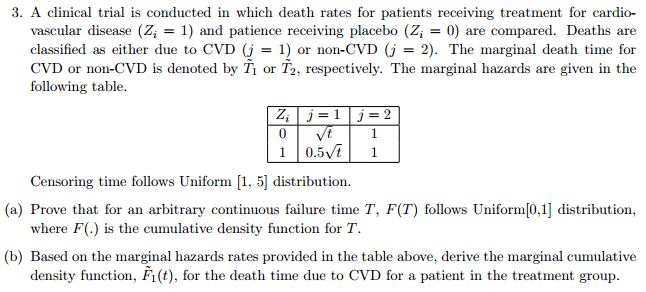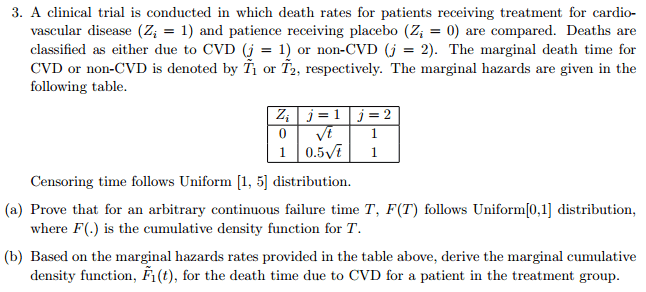# Survival Analysis: find marginal CDF from marginal hazard rates?

NewNameStat

## Homework Statement## Homework Equations

My professor gives us the following formula: [ tex ] F_j(t) = \int\limits_0^t exp\Big\{-\sum\limits_{j=1}^J \int\limits_0^u \lambda_j^{\#}(v)dv \Big\} \lambda_j^{\#}(u)du[ / tex ] where [ tex ]\lambda_j^{\#}(t) [ / tex ] are the cause-specific hazard rates for cause $j$.

## The Attempt at a Solution

Assuming we have independent competing risks (we have not yet talked about dependent competing risks so I think it's safe to assume this), $\tilde{F}_j(t) = \int\limits_0^t exp\Big\{-\sum\limits_{j=1}^J \int\limits_0^u \tilde{\lambda}_j(v)dv \Big\} \tilde{\lambda}_j(u)du$ where $\tilde{\lambda}_j(u)$ is the marginal hazard rate for cause $j$.

So, $\tilde{F}_1(t) = \int\limits_0^t exp\Big\{- \int\limits_0^u 1 dv - \int\limits_0^u .5\sqrt{v} \; dv \Big\} .5\sqrt{u} \; du = .5\int\limits_0^t exp\Big\{- u - \frac{1}{3}u^{3/2}\; dv \Big\} \sqrt{u}\;du$.

However, I do not think this is right because $\tilde{F}_1(\infty)\neq 1$ (it equals something around $0.25$).

Homework Helper
Dearly Missed

## Homework Statement## Homework Equations

My professor gives us the following formula: [ tex ] F_j(t) = \int\limits_0^t exp\Big\{-\sum\limits_{j=1}^J \int\limits_0^u \lambda_j^{\#}(v)dv \Big\} \lambda_j^{\#}(u)du[ / tex ] where [ tex ]\lambda_j^{\#}(t) [ / tex ] are the cause-specific hazard rates for cause $j$.

## The Attempt at a Solution

Assuming we have independent competing risks (we have not yet talked about dependent competing risks so I think it's safe to assume this), $\tilde{F}_j(t) = \int\limits_0^t exp\Big\{-\sum\limits_{j=1}^J \int\limits_0^u \tilde{\lambda}_j(v)dv \Big\} \tilde{\lambda}_j(u)du$ where $\tilde{\lambda}_j(u)$ is the marginal hazard rate for cause $j$.

So, $\tilde{F}_1(t) = \int\limits_0^t exp\Big\{- \int\limits_0^u 1 dv - \int\limits_0^u .5\sqrt{v} \; dv \Big\} .5\sqrt{u} \; du = .5\int\limits_0^t exp\Big\{- u - \frac{1}{3}u^{3/2}\; dv \Big\} \sqrt{u}\;du$.

However, I do not think this is right because $\tilde{F}_1(\infty)\neq 1$ (it equals something around $0.25$).

## The Attempt at a Solution

I have re-formatted your tex commands, to make them work properly. You should:
(1) remove the spaces in "[ tex ]" and " [ / tex ]", writing "[TEX]" and "[/TEX]" (but all in lower-case letters); and
(2) use "\exp" instead of "exp".
Also: removing the superscript # from the lambdas would make it look better; those superscripts serve no useful purpose. Just writing ##\lambda_j## is good enough. However, I did not do that, since what you wrote is not incorrect---just unnecessary.

$$F_j(t) = \int\limits_0^t \exp\Big\{-\sum\limits_{j=1}^J \int\limits_0^u \lambda_j^{\#}(v)dv \Big\} \lambda_j^{\#}(u)du$$

Finally: I do not understand the above formula. If, say, there were 1000 patients involved and each treatment involved 500 patients, the probability that a randomly-chosen patient falls in class 1 or class 2 is 1/2, giving F1 as a mixture:
$$F_1(t) = \frac{1}{2} F_{11}(t)+\frac{1}{2} F_{12}(t).$$
However, if unequal numbers got treatments 1 and 2, such as 400 receiving treatment 1 and 600 receiving treatment 2, we should have
$$F_1(t) = \frac{4}{10} F_{11}(t) + \frac{6}{10} F_{12}(t).$$
Here, ##F_{11}## uses ##\lambda_1 = \sqrt{t}## and ##F_{12}## uses ##\lambda_2 = 0.5\sqrt{t}##.

I did not even attempt to read your analysis, since the tex commands are not working; please edit and re-submit.